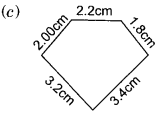# DAV Class 6 Maths Chapter 15 Worksheet 1 Solutions

The DAV Class 6 Maths Book Solutions and DAV Class 6 Maths Chapter 15 Worksheet 1 Solutions of Perimeter and Area offer comprehensive answers to textbook questions.

## DAV Class 6 Maths Ch 15 WS 1 Solutions

Question 1.
A field is in the shape of a rectangle whose length is 130 m and breadth is 95 m respectively. Find the cost of fencing the field at ₹ 20 per metre.
The perimeter of the rectangle
= 2[l + 6]
= 2[130 m + 95 m]
= 2 × [225 m]
= 450 m
Cost of fencing = ₹ 20 × 450
= ₹ 9000
Hence the required cost = ₹ 9000.

Question 2.
Akshay covers a rectangular field three times whose length and breadth are 25 m and 19 m respectively. How much distance does he cover?
Perimeter of the field = 2[l + b]
= 2[25 m + 19 m]
= 2 × 44 m
= 88 m
Total Distance covered by Akshay = 3 × 88 m = 264 m
Hence the required distance = 264 m.Question 3.
The length of one side of a square field is 25 m. Find the cost of fencing at the rate of ? 18 per metre.
The perimeter of a square = 4 × side = 4 × 25 = 100 m
Cost of fencing = ₹ 100 × 18 = ₹ 1800
Hence the required cost = ₹ 1800

Question 4.
Raju can round a rectangular garden of length 30 m and breadth 15 m. Manu ran around a square field of side 35 m. Who covered more distance and by how much?
Perimeter of a rectangle = 2 [l + b]
= 2[30 m + 15 m]
= 2 × 45 m = 90 m.
∴ The distance covered by Raju in one round = 90 m.
The distance covered by Manu in round = 4 × 35 = 140 m
∴ Manu covered more distance by 140 m – 90 m = 50 m.

Question 5.
Vineet wants to polish the floor of a room which is 140 cm long and 120 cm wide. The charges for polishing the floor is ₹ 52 per square metre. What will be the cost of polishing the floor of the room?
Area of the rectangle
= length × breadth = 140 cm × 120 cm
= 16800 cm2 = 16800 m2 + 10000
= 1.68 m2
Charge for polishing the floor = ₹ (1.68 × 52) = ₹ 87.36
Hence the required cost = ₹ 87.36

Question 6.
A carpet is 6 m long and 4.5 m wide. Find the area of the carpet. Also find the cost of the carpet if it costs ? 45 per square metre.
Area of the carpet = l × b
= 6 m × 4.5 m = 27 m2
Cost of the carpet = ₹(27 × 45)
= ₹ 1215
Hence the required cost = ₹ 1215.

Question 7.
A square park is to be watered. If one side of the park is 4.2 m, find the area to be watered.
Side of the square park = 4.2 m
∴ Area of the park = (4.2 × 4.2) m2 = 17.64 m2
Hence the required area = 17.64 m2Question 8.
Which playground has bigger area – one that measures -50 m by 40 m or the other that measures 75 m by 20 m?
Area of the first playground = l × b
= 50 m × 40 m
= 2000 m2

Area of the second playground = l × b
= 75 m × 20 m
= 1500 m2
Hence the first playground has bigger area.

### DAV Class 6 Maths Chapter 15 Worksheet 1 Notes

1. Perimeter of rectangle = 2 × [length + breadth]2. Perimeter of square = 4 × side
3. Area of rectangle = length × breadth.
4. Area of square = (Side)2

Example 1:
Find the perimeter of the following figures:Solution:
Perimeter of rectangle = 2 [l + b]
= 2[2.4 + 1.8]
= 2 × 4.2
= 8.4 cmSolution:
= 3.6 cm + 2.4 cm + 1.8 cm + 3.00 cm
= 10.8 cmSolution:
Perimeter of the pentagon
= 3.2 cm + 3.4 cm + 1.8 cm + 2.2 cm + 2.00 cm
= 12.6 cmSolution:
Perimeter of the pentagon
= 4.2 cm + 2.4 cm + 2.2 cm + 4.2 cm + 2.8 cm
= 15.8 cm

Example 2:
If the perimeter of square is 32 cm, find its side.
Solution:
Perimeter of square = 4 × side
∴ Side = $$\frac{1}{4}$$ × Perimeter
= $$\frac{1}{4}$$ × 32 = 8 cm
Hence the side = 8 cm.

Example 3:
Find the perimeter of a rectangle if its length is 16 m and breadth 12 m.
Solution:
Length = 16 m,Breadth = 12 m
. .Perimeter of the rectangle= 2[l + b]
= 2[16 m + 12 m]
= 2 × 28 m = 56 m
Hence the perimeter of the rectangle is 56 m.Example 4:
A lawn is 200 m long and 150 m wide. The gardener wants a fencing of barbbed wire all around it. Find the cost of the wire at the rate of ₹ 20 per metre.
Solution:
Perimeter of the lawn = 2 [l + b]
= 2 [200 m + 150 m]
= 2 × 350 m
= 700 m
Cost of barbbed wire = ₹ 700 × 20
= ₹ 14000
Hence the total cost = ₹ 14000

Example 5:
Find the area of a rectangular field having 20 m length and 15 m 75 cm breadth.
Solution:
Area of rectangle = length × breadth
= (20 × 15.75) m2
= 315 m2
Hence the required area = 315 m2